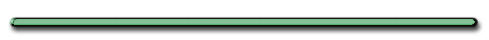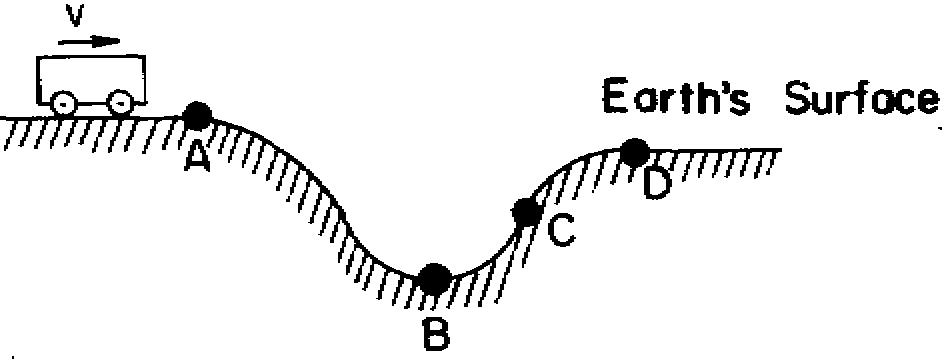HomeVideo Instruction

showmethephysics.com

 Ex 2) A 10 kg frictionless cart is resting on a horizontal table.  A force of 10 N is applied to the cart for a distance of 8 m. What is the carts new KE?

Ex 2) A 10 kg frictionless cart is resting on a horizontal table.  A force of 10 N is applied to the cart for a distance of 8 m. What is the carts new kinetic energy?

m = 10 kg

F = 10 N

d = 8 m

KE = (½)mv??

ΔKE = W

= Fd = 10N(8m)

= 80 Nm

 Ex 3) 100 Joules of work is needed to lift a 100 kg object from the ground.   What is the objects new P.E.?Ex 3) 100 Joules of work is needed to lift a 100 kg object from the ground.What is the objects new P.E. ?

W =
ΔPE = 100J

 Ex 4) Crate is pulled 6.00 m up an incline with a force of 50.0 N. P.E. of  cart increases by 250. J. Total work done against friction?

Ex 4) Crate is pulled 6.00 m up an incline with a force of 50.0 N.  If the PE of the cart increases by 250. J. , what is the total work done against friction?

d = 6.00 m

F = 50.0 N

ΔPE = 250. J

W = Fd = 50.0 N(6 m)

= 300. J

W = ΔPE + Wf

300. J = 250. J + Wf

Wf = 50.0 J

 Ex 5) 4 N is exerted on a 1 kg mass at rest for 2 m, causing it to move   A. Find the objects K.E.

KE = (1/2)mv2

Work on object = ΔKE

ΔKE = W = Fd = 4 N(2 m)

= 8 Nm

B. Find the objects velocity

KE = (1/2)mv2

8 Nm = (1/2)1 kg(v2)

16 = v2

V = 4 m/s

 Ex 6) A force of 100.0 N lifts the object 10.00 m off the ground.  What is the object's new PE? What is the mass of the object?Ex 6) A force of 100.0 N lifts the object 10.00 m off the ground.  What is the object's new potential energy?

 mass of object?ΔPE = W = Fd

= 100.0 N(10.00 m)

= 1000. Nm

What is the mass of the object?

PE = 1000. J = mgh

= m(9.8 m/s2)10.00 m = 1000. J

m = 10.20 kg

 Ex 7) Spring has a spring constant of 2.0 N/m.   How much work must be done to stretch 5.0 m from its equilibrium position?Ex 7) A spring has a spring constant of 2.0 N/m. How much work must be done to stretch it 5.0 m from its equilibrium position?

 k = 2.0 N/m x = 5.0 m W = ?W = PE = 1/2kx2

= (1/2)2.0 N/m(5.0 m)2

= 25. J

 RankingTaskRank the KE of the car from largest to smallest         B, C, D, A

 How much farther will a car traveling at 100 km/s skid than the same car traveling at 50 km/s? A) 4X as far B) 2 X as far C) The same distance D) 5X as far E) 1/2 as far

Review

Name the energy conversion that
occurs in a Grandfather clock.

PE of the hanging weights
converts to the KE of the clockStatic ElectricityEnrichment

Superfluids

The strange behavior of liquid helium

BBC